Test Description

## 15 Questions MCQ Test Science Olympiad Class 7 | Olympiad Test: Dice

Olympiad Test: Dice for Class 7 2023 is part of Science Olympiad Class 7 preparation. The Olympiad Test: Dice questions and answers have been prepared according to the Class 7 exam syllabus.The Olympiad Test: Dice MCQs are made for Class 7 2023 Exam. Find important definitions, questions, notes, meanings, examples, exercises, MCQs and online tests for Olympiad Test: Dice below.
 1 Crore+ students have signed up on EduRev. Have you?
Olympiad Test: Dice - Question 1

### The positions of a cube are shown below, Which letter will be on the face opposite to face with ‘A’?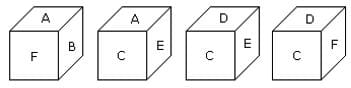Detailed Solution for Olympiad Test: Dice - Question 1

The letters of the adjacent faces to the face with letter A are B, F, C and E. Hence D is the letter of the face opposite to the face with letter (A).

Olympiad Test: Dice - Question 2

### Two positions of a dice are shown below. When 3 points are at the bottom, how many points will be at the top?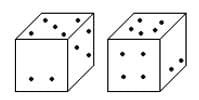Detailed Solution for Olympiad Test: Dice - Question 2

According to the rule (2), when 3 points are at the bottom then 4 points will be at the top.

Olympiad Test: Dice - Question 3

### Observe the dots on the dice (one to six dots) in the following figures. How many dots are contained on the face opposite to the face containing four dots?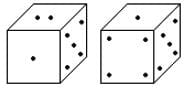Detailed Solution for Olympiad Test: Dice - Question 3

Here one of the two common faces (5) is in the same position, then according to the rule (2), the remaining face with the 4 dots will be opposite to face with dots 2.

Olympiad Test: Dice - Question 4

How many points will be on the face opposite to the face which contains 3 points?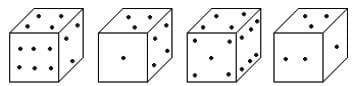Detailed Solution for Olympiad Test: Dice - Question 4

The adjacent faces to the face which 3 points have 2, 1, 4 and 6 points. Hence on the face which is opposite to the face which contains 3 points, there will be 5 points.

Olympiad Test: Dice - Question 5

When the digit 5 is on the bottom, which number will be on its upper surface?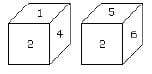Detailed Solution for Olympiad Test: Dice - Question 5

According to the rule (3), common faces with number 2 are in same positions. Hence when the digit 5 is on the bottom, 1 will on the upper surface.

Olympiad Test: Dice - Question 6

From the four positions of a dice given below, find the colour which is opposite to yellow?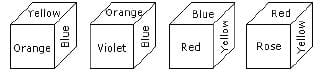Detailed Solution for Olympiad Test: Dice - Question 6

The colours adjacent to yellow are orange, blue, red and rose. Hence violet will be opposite to yellow.

Olympiad Test: Dice - Question 7

Two positions of a cubical block are shown. When 5 is at the top, which number will be at bottom?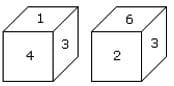Detailed Solution for Olympiad Test: Dice - Question 7

In these 2 positions one common face with number 3 is in the same position. Hence according to rule (3), 1 is opposite to 6 and 4 is opposite to 2. Therefore 5 is opposite to 3.

Olympiad Test: Dice - Question 8

Here 4 positions of a cube are shown. Which sign will be opposite to ‘+’?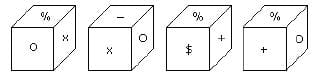Detailed Solution for Olympiad Test: Dice - Question 8

From position I and III common face with % is in the same position. Hence according to rule (3) opposite is x.

Olympiad Test: Dice - Question 9

Two positions of a dice are shown below. How many points will be on the top when 2 points are at the bottom?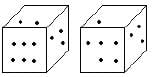Detailed Solution for Olympiad Test: Dice - Question 9

In these 2 positions of a dice, one common face having points 3 is in the same position. Hence, according to rule (3), there will be 4 points on the required face.

Olympiad Test: Dice - Question 10

Here two positions of a dice are shown. If there are two dots in the bottom, then how many dots will be on the top?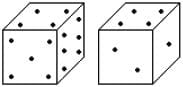Detailed Solution for Olympiad Test: Dice - Question 10

Here the common faces with 4 dots are in the same positions.
Hence 2 will be opposite to 5.

Olympiad Test: Dice - Question 11

Two positions of a cube with its numbered surfaces are shown below. When the surface 4 touches the bottom, which surface will be on the top?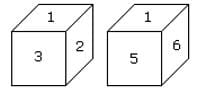Detailed Solution for Olympiad Test: Dice - Question 11

In these 2 positions one common face with number 1 is in the same position. Hence according to the rule number (3), 2 is opposite 6 and 3 is opposite to 5. Therefore opposite to 4 is 1.

Olympiad Test: Dice - Question 12

Two positions of a dice are shown below. When number ‘1’ is on the top. What number will be at the bottom?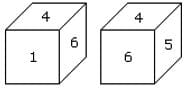Detailed Solution for Olympiad Test: Dice - Question 12

According to the rule (2) when ‘one’ is at the top, then 5 will be at the bottom.

Olympiad Test: Dice - Question 13

Which number is on the face opposite to 6?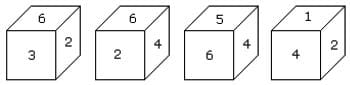Detailed Solution for Olympiad Test: Dice - Question 13

As the numbers 2, 3, 4 and 5 are adjacent to 6. Hence the number on the face opposite to 6 is 1.

Olympiad Test: Dice - Question 14

Six dice with upper faces erased are shown as:

The sum of the numbers of dots on the opposite face is 7.

Q. ​If even numbered dice have even number of dots on their top faces, then what would be the total number of dots on the top faces of their dice?

Detailed Solution for Olympiad Test: Dice - Question 14

Even numbered dice are: (II), (IV) and (VI)
No. of dots on the top face of (II) dice = 6 No. of dots on the top face of (IV) dice = 6 and No. of dots on the top face of (VI) dice = 6 Therefore required total = 6 + 6 + 6 = 18

Olympiad Test: Dice - Question 15

How many points will be on the face opposite to the face which contains 2 points?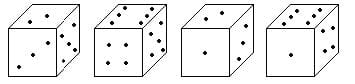Detailed Solution for Olympiad Test: Dice - Question 15

In first two positions of dice one common face containing 5 is same. Therefore according to Rule no. (3) the face opposite to the face which contains 2 point will contain 6 points.
A cube has six different symbols drawn over its six faces. The symbols are dot, circle, triangle, square, cross and arrow. Three different positions of the cube are shown in figures X, Y, and Z.